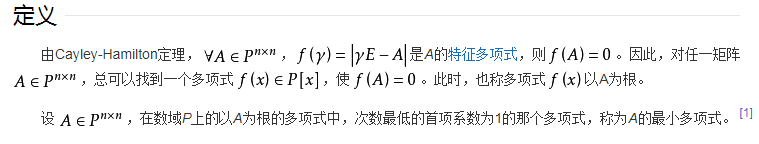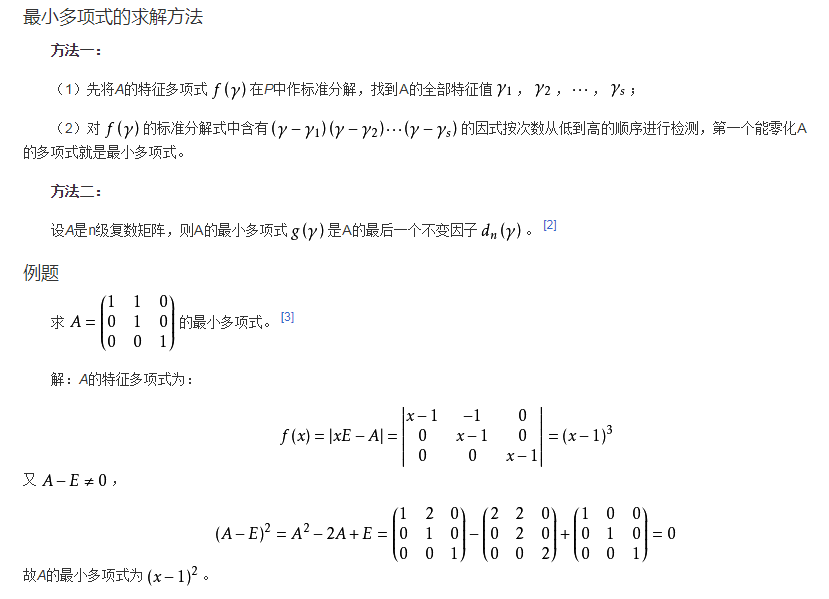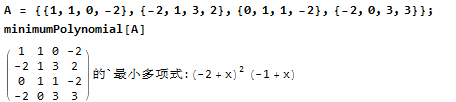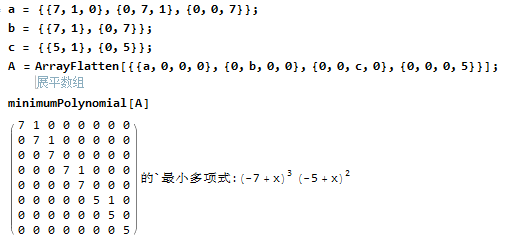# 最小多项式计算2018年3月7日06:18:31

2 1340字阅读4分28秒

## 最小多项式介绍## Mathematica实现最小多项式计算

``````matrixhorner[cl_, m_] :=  Module[{n = Length[cl], k = Length[m], id, pm},
id = IdentityMatrix[k];
pm = cl[[-1]] id;
Do[
pm = cl[[j]] id + m.pm,
{j, n - 1, 1, -1}];
pm];

minimumPolynomial[A_] := Block[{nlList, nlExp, nNlExp, FA},
nlList = FactorList[CharacteristicPolynomial[A, x]];
nlExp = nlList[[#, 2]] & /@ Range@Length[nlList];
nNlExp = Tuples[Range[nlExp[[#]]] & /@ Range[Length[nlExp]]];
Do[
nlList[[All, 2]] = nNlExp[[i]];
FA = Times @@ Power @@@ nlList;
If[matrixhorner[CoefficientList[FA, x], A] ==  ConstantArray[0, {Length[A], Length[A]}], Print[TraditionalForm[A], "的`最小多项式:", FA]; Break[]];
, {i, 1, Length[nNlExp]}]
]``````

``````A = {{1, 1, 0, -2}, {-2, 1, 3, 2}, {0, 1, 1, -2}, {-2, 0, 3, 3}};
minimumPolynomial[A]````````````a = {{1, 1, 0, -2}, {-2, 1, 3, 2}, {0, 1, 1, -2}, {-2, 0, 3, 3}};
(a - IdentityMatrix).MatrixPower[(a - 2*IdentityMatrix),  2] // MatrixForm``````

``````a = {{7, 1, 0}, {0, 7, 1}, {0, 0, 7}};
b = {{7, 1}, {0, 7}};
c = {{5, 1}, {0, 5}};
A = ArrayFlatten[{{a, 0, 0, 0}, {0, b, 0, 0}, {0, 0, c, 0}, {0, 0, 0, 5}}];
minimumPolynomial[A]``````• 微信公众号
• 关注微信公众号
•• QQ群
• 我们的QQ群号
•• 本文由 发表于 2018年3月7日06:18:31
• 转载请务必保留本文链接：https://mathpretty.com/9052.html